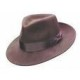# convert the yaw Euler angle into into the range [0 , 360].

Hi everyone,

I used the following code to get the yaw Euler angle:

// Convert quaternion to RPY.
tf::Quaternion q;
tf::quaternionMsgToTF(imu_msg->orientation, q);
tf::Matrix3x3(q).getRPY(roll, pitch, yaw);


I get the Euler angle varying from [0,PI] and [-PI, 0]

I need rotation angles in the range of 0 to 360. Is it possible to get that directly?

edit retag close merge delete

Sort by » oldest newest most votedYou can't get angles in degrees directly from the available library calls, but it's pretty trivial to convert radians to degrees:

double yaw = ...; // however you want to get yaw.
double yaw_degrees = yaw * 180.0 / M_PI; // conversion to degrees
if( yaw_degrees < 0 ) yaw_degrees += 360.0; // convert negative to positive angles

more

This answer was some time ago, but is this not incorrect?

PEP 103 specifies that yaw measured from the x-axis (i.e. in a geographic frame from East) and is positive CCW. Heading of course is measured from the y-axis, positive CW.You can try the angles package which provides C++ api calls for angle normalization, conversions between radians and degrees, and finding minimum angular distance.

more

thanks for your answer, please can you tell me how to use the angles package? I am not succeed.

Without more info about how you are not succeeding, it's hard to help. You need to (1) include angles in your manifest.xml or package.xml (2) #include <angles angles.h=""> (3) Run code like "yaw = angles::to_degrees(angles::normalize_angle_positive(yaw));"

Sorry for the bad formatting of point (2).... not my fault. See [bug report](https://github.com/ros-infrastructure/answers.ros.org/issues/65)